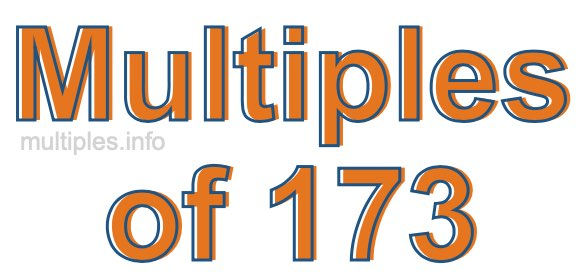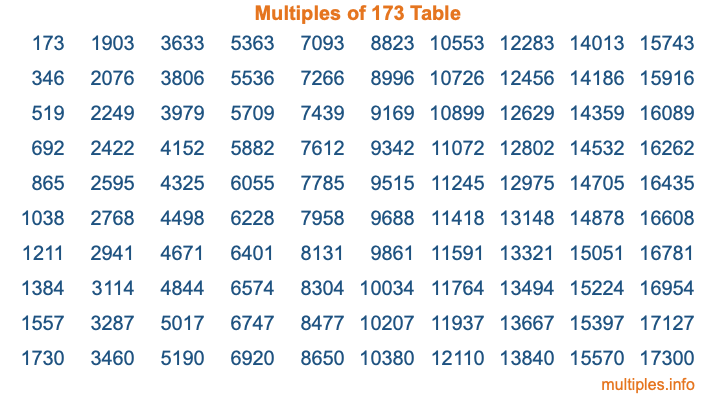Multiples of 173Welcome to the Multiples of 173 page. Here we will first teach you everything you will ever need to know about the multiples of 173, and then give you a study guide summary of everything we taught you to make sure you remember it all. Use this page to look up facts and learn information about the multiples of 173. This page will make you a multiples of one hundred seventy-three expert!

Definition of Multiples of 173
Multiples of 173 are all the numbers that when divided by 173 equal an integer. Each of the multiples of 173 are called a multiple. A multiple of 173 is created by multiplying 173 by an integer.

Therefore, to create a list of multiples of 173, you start with 1 multiplied by 173, then 2 multiplied by 173, then 3 multiplied by 173, and so on for as long as you want. Thus, the list of the first five multiples of 173 is 173, 346, 519, 692, and 865. To see a larger list of multiples of 173, see the printable image of Multiples of 173 further down on this page. We also have a category where you can choose any nth multiple of 173.

Multiples of 173 Checker
The Multiples of 173 Checker below checks to see if any number of your choice is a multiple of 173. In other words, it checks to see if there is any number (integer) that when multiplied by 173 will equal your number. To do that, we divide your number by 173. If the the quotient is an integer, then your number is a multiple of 173.

Is  a multiple of 173?

Least Common Multiple of 173 and ...
A Least Common Multiple (LCM) is the lowest multiple that two or more numbers have in common. This is also called the smallest common multiple or lowest common multiple and is useful to know when you are adding our subtracting fractions. Enter one or more numbers below (173 is already entered) to find the LCM.

Check out our LCM Calculator if you need more details about the Least Common Multiple or if you need the LCM for different numbers for adding and subtraction fractions.

nth Multiple of 173
As we stated above, 173 is the first multiple of 173, 346 is the second multiple of 173, 519 is the third multiple of 173, and so on. Enter a number below to find the nth multiple of 173.

th multiple of 173

Multiples of 173 vs Factors of 173
173 is a multiple of 173 and a factor of 173, but that is where the similarities end. All postive multiples of 173 are 173 or greater than 173. All positive factors of 173 are 173 or less than 173.

Below is the beginning list of multiples of 173 and the factors of 173 so you can compare:

Multiples of 173: 173, 346, 519, 692, 865, etc.

Factors of 173: 1, 173

As you can see, the multiples of 173 are all the numbers that you can divide by 173 to get a whole number. The factors of 173, on the other hand, are all the whole numbers that you can multiply by another whole number to get 173.

It's also interesting to note that if a number (x) is a factor of 173, then 173 will also be a multiple of that number (x).

Multiples of 173 vs Divisors of 173
The divisors of 173 are all the integers that 173 can be divided by evenly. Below is a list of the divisors of 173.

Divisors of 173: 1, 173

The interesting thing to note here is that if you take any multiple of 173 and divide it by a divisor of 173, you will see that the quotient is an integer.

Multiples of 173 Table
Below is an image of the first 100 multiples of 173 in a table. The table is in chronological order, column by column. The first column has the first ten multiples of 173, the second column has the next ten multiples of 173, and so on.The Multiples of 173 Table is also referred to as the 173 Times Table or Times Table of 173. You are welcome to print out our table for your studies.

Negative Multiples of 173
Although not often discussed or needed in math, it is worth mentioning that you can make a list of negative multiples of 173 by multiplying 173 by -1, then by -2, then by -3, and so on, to get the following list of negative multiples of 173:

-173, -346, -519, -692, -865, etc.

Multiples of 173 Summary
Below is a summary of important Multiples of 173 facts that we have discussed on this page. To retain the knowledge on this page, we recommend that you read through the summary and explain to yourself or a study partner why they hold true.

There are an infinite number of multiples of 173.

A multiple of 173 divided by 173 will equal a whole number.

173 divided by a factor of 173 equals a divisor of 173.

The nth multiple of 173 is n times 173.

The largest factor of 173 is equal to the first positive multiple of 173.

173 is a multiple of every factor of 173.

173 is a multiple of 173.

A multiple of 173 divided by a divisor of 173 equals an integer.

173 divided by a divisor of 173 equals a factor of 173.

Any integer times 173 will equal a multiple of 173.

Multiples of a Number
Here you can get the multiples of another number, all with the same attention to detail as we did for multiples of 173 on this page.

Multiples of
Multiples of 174
Did you find our page about multiples of one hundred seventy-three educational? Do you want more knowledge? Check out the multiples of the next number on our list!